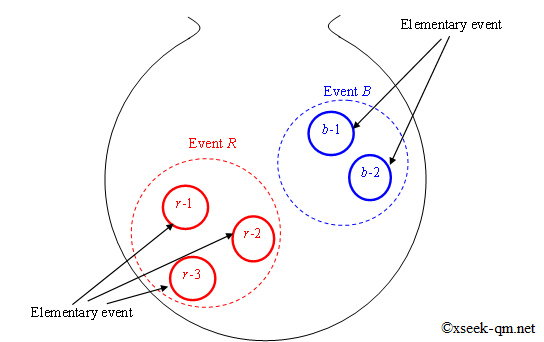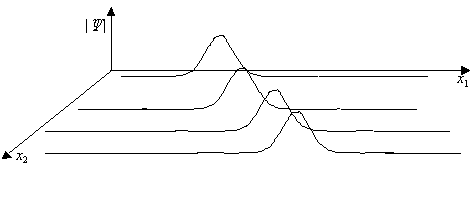# Derivation of Born rule of quantum mechanics

2014/12/23
Published 2012/9/26

In the Born rule of quantum mechanics, we interpret the wave function of a certain electron as the observation probability of that electron. Born rule is that the observation probability of small particles like electrons is proportional to the square of the absolute value of the particle's wave function. This page introduces the method of deriving Born rule of quantum mechanics.

## Is Born rule the basic principle?

In general, Born rule is considered to be basic principle of quantum mechanics. The basic principle means that it is a premise that can not be questioned any more. Therefore, many people do not think to derive Born rule from another basic principle. So why am I trying to derive Born rule from another basic principle?

That is because I have the question "Is Born rule the basic principle?" According to Born rule, the observation probability of electrons is proportional to the square of the absolute value of the wave function. I do not think that Born rule is the basic principle. Because I think that probability should be calculated with Laplace probability theory. The probability theory of Laplace is explained in the next section.

## Laplace's probability theory

Laplace probability theory is the theory to calculate the probability. French mathematician Pierre-Simon Laplace proposed its theory in 1814. For example, suppose you have three red balls and two blue balls in a bag.Probability theory of Laplace

Put your hands in the bag and take out the ball. What is the probability of taking red balls? This probability can be calculated by Laplace probability theory. In Laplace probability theory, we use the concept of events and elementary events. A elementary event is an event that can not be further divided. For example, the event R of picking out red spheres can be divided into the following three kinds of events.

1. Event to take out the sphere r-1
2. Event to take out the sphere r-2
3. Event to take out the sphere r-3

'Event to take out the sphere r-1' is an elementary event as it can not be further divided. In Laplace probability theory, we calculate the probability by dividing the number of expected elementary events by the number of all elementary events. For example, the probability of an event R to extract a red sphere is 3/5. Because the number of expected elementary events is 3 and the number of all elementary events is 5.

The method of calculating the probability based on Born's rule is entirely different from the calculation method based on Laplace's probability theory. In Laplace 's probability theory, why calculate the probability by the square of the absolute value of the wave function in Born' s rule, to calculate the probability by the ratio of the number of elementary events? On this page we will call this question "probability problem of quantum mechanics". On this page, we will consider solution of this probability problem with many-world interpretation and probability theory. In the next section we will explain the many-world interpretation.

## Many-world interpretation by many-particle wave function

In many-world interpretation, we express everything in the world by wave function. The image for this "wave function expressing all of the world" differs greatly among individuals. In order to imagine the wave function of the universe, it is necessary to gradually increase the number starting from the wave function of one particle.

In order to think about the probability problem, we consider the wave function of many-particle.

Assuming that the position of the k- th elementary particle is \begin{align*} r_k=(x_k,y_k,z_k), \end{align*} the wave function of the many-particle becomes as follows.

\begin{align*} \Psi=\Psi(r_1,r_2,r_3,\cdots,r_n) \end{align*}

This wave function expresses the position of n elementary particles. If you change the arrangement of elementary particles, you can construct any universe.Many-particle wave function

One point of the wave function of many-particle corresponds to one world. The probability distribution P is as follows.

\begin{align*} P(r_1,r_2,r_3,\cdots,r_n)=|\Psi(r_1,r_2,r_3,\cdots,r_n)|^2 \end{align*}

## Derivation of Born's rules by many-world interpretation and probability theory

The following paper derives Born 's rules with many - world interpretation and probability theory.

In deriving the Born rules in a many-world interpretation, the concept that is needed is the concept of the next hierarchical universe. For details of the hierarchical universe, please refer to the paper.Hierarchical universe

## Hierarchical universe

Here I will explain the outline of the hierarchical universe.

The hierarchical universe is a nested universe.

There is a particle in our universe. And there is a wave function corresponding to that particle. I think that there is another small universe whose radius is the absolute value of that wave function.

There is a particle in the small universe. And there is a wave function corresponding to that particle. I think that there is another smaller universe whose radius is the absolute value of the wave function.

There is also a wave function whose absolute value is the radius of our universe. There is a particle corresponding to that wave function. That particle exists in another big universe.

In other words, our universe and the small universe are connected by a wave function.

Related articles: